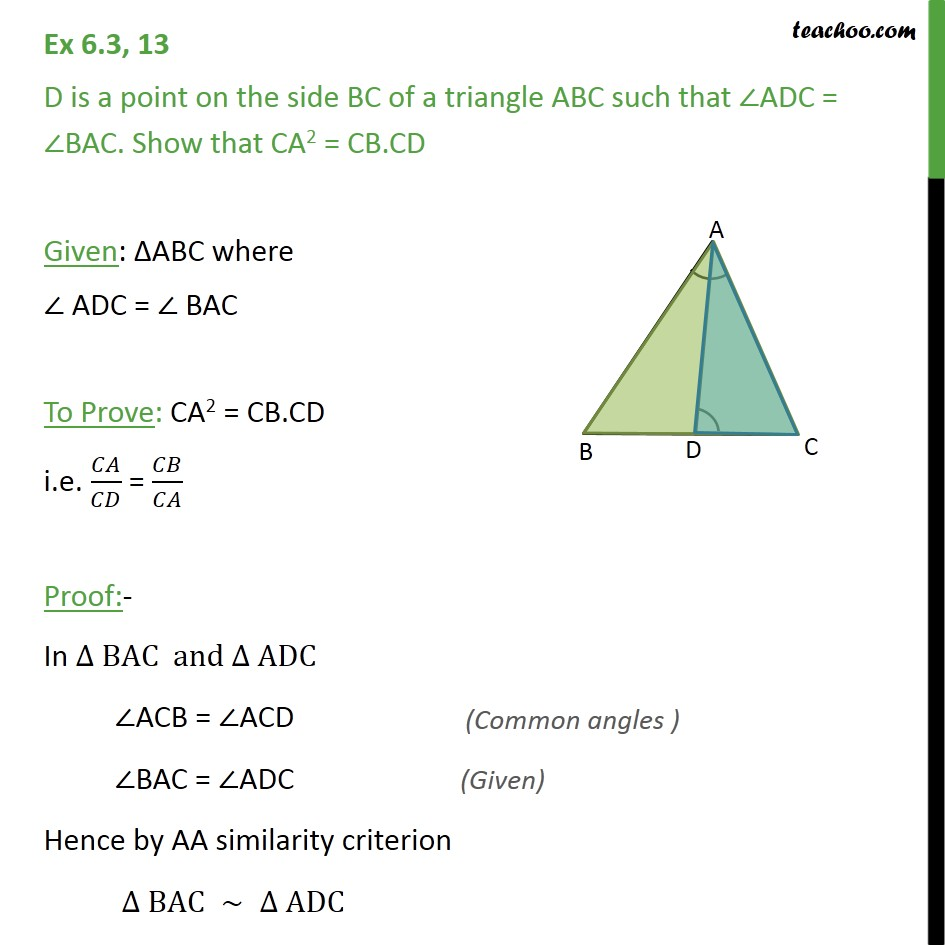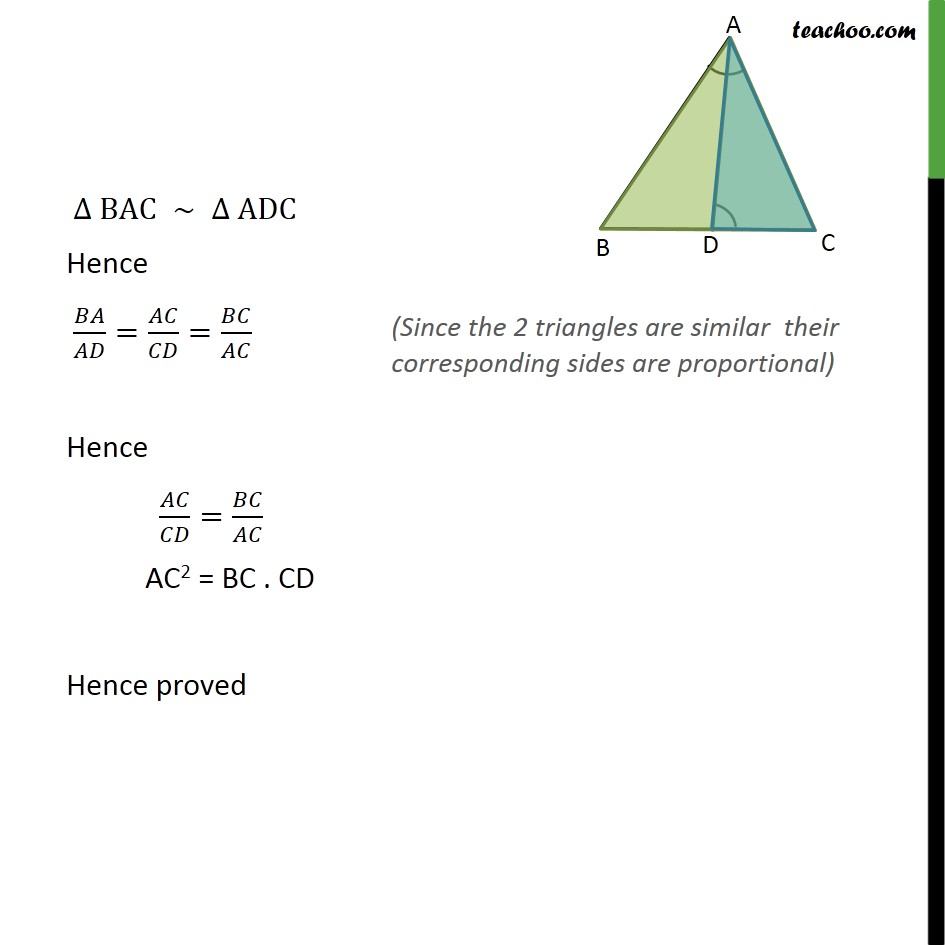1. Chapter 6 Class 10 Triangles
2. Concept wise
3. AA Similarity

Transcript

Ex 6.3, 13 D is a point on the side BC of a triangle ABC such that ADC = BAC. Show that CA2 = CB.CD Given: ABC where ADC = BAC To Prove: CA2 = CB.CD i.e. / = / Proof:- In BAC and ADC ACB = ACD BAC = ADC Hence by AA similarity criterion BAC ADC BAC ADC Hence / = / = / Hence / = / AC2 = BC . CD Hence proved

AA Similarity